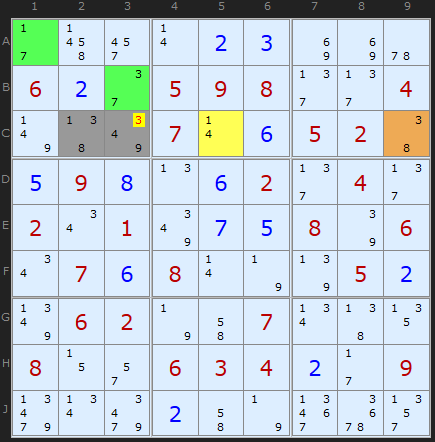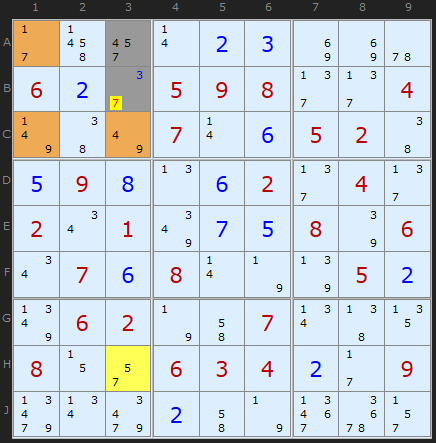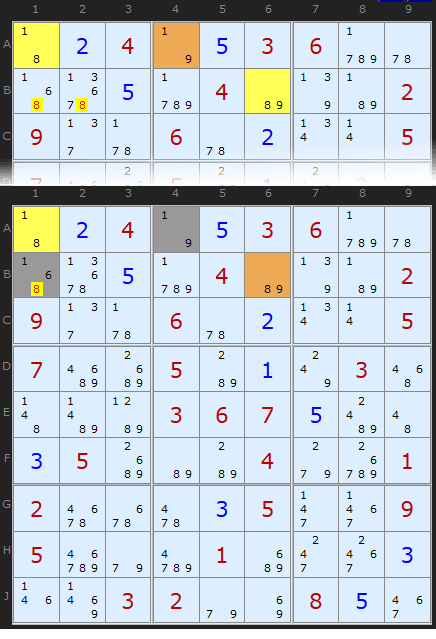Main Page - Back

# Aligned Pair Exclusion

From sudokuwiki.org, the puzzle solver's siteThis is an interesting strategy, known by the short-hand as APE and sometimes called Subset Exclusion. It can overlap with Y-Wings, XYZ-Wings and WXYZ-Wings but uses very different logic. The overlap is not strict so they are worth looking out for in a tough situation.

There is always a base pair of cells (which now show up as grey cell on the solver). At least one elimination will occur in one of those two cells. The solver will also show a variety of colored cells which are the elements used to make an elimination. I used to distinguish between APE type 1 which only used bi-value cells and type 2 which used 2-cell Almost Locked Sets (ALSs). But the solver will now find a larger variety including 3-cell ALSs and since these merely extend the same logic the solver will return the first of any it finds. A better Type 1 and Type 2 distinction is between the base pair of cells which can be a locked pair (ie can see each other) or not (can't see each other). The logic is subtly different but I'll come to this in the following examples.

## Aligned Pair Exclusion - Type 1

The Aligned Pair Exclusion can be succinctly stated: Any two cells that can see each other CANNOT duplicate the contents of any Almost Locked Set they both entirely see and share candidates with.

Remember - a bi-value cell (with two candidates) is the simplest Almost Locked Set since it is a set of size '1' with size+1 (ie two) candidates.

Let's consider the simplest possible example - two bi-value cell attacking the pair. I have also shown the Y-Wing in the diagram so we can see there is a simpler way to do the same job - but only in some cases.

We consider ALL the possible pairs of numbers that will fit in [G2/G3]. These are for G2 and G3:

2 and 2 (impossible)
2 and 5
2 and 8
4 and 2
4 and 5
4 and 8

Apart from the first being impossible (2 and 2) since G2 and G3 can see each other, we have problems with some of the other combinations. What if 2 and 8 were tried as the solutions? Well, that would duplicate and therefore empty G9. Also 4 and 8 would empty H1.
We are left with a set of combinations that looks like this:

2 and 2 (impossible)
2 and 5
2 and 8 (impossible)
4 and 2
4 and 5
4 and 8 (impossible)

Notice that we now have no 8 left in any pairing? Therefore we can remove 8 from our base pair. Voilà

## Example 2APE example 2 : Load Example or : From the Start The next example has tri-values spread over two cells as part of the attack. The way we can use double cells is by saying that any two cells with only abc excludes combinations ab, ac and bc from the base pair under consideration. This neat trick greatly extends the usefulness of APE which would otherwise be a just poor man's Y-Wing.

The 2-cell ALS in [A1,B3] contains {1/3/7} so pairs that would cripple the solution for that ALS are {1,3}, {1,7} and {3,7}.

Let's consider all the possible pairs of numbers in our base pair [C2/C3]. These are:

1 and 3 - excluded by A1 + B3
1 and 4 - excluded by C5
1 and 9
3 and 3
3 and 4
3 and 9
8 and 3 - excluded by C9
8 and 4
8 and 9

Now, we have to be a tiny bit careful here. 3 has definitely been excluded as a possible solution in C3 but look down the list and 3 + 4 is still OK and 3 + 9 is OK. So we can't remove 3 from C2 just yet.

Credits: Myth Jellies came up with the insight for abc = ab/ac/bc

Note: There could be more than two, sometimes three or four ALSs of several sizes in an APE attack. I've considered examples with two for simplicity's sake

## Example 3APE Example 3 : Load Example or : From the Start Further into the same puzzle we come across a 3-cell ALS plus a bi-value in H3 attacking A3/B3. The ALS in [A1,C1,C3] contains the four numbers {1,4,7,9} which the solver thinks of as a quadruple combination. The combinations of 'abcd' are ab, ac, ad, bc, bd and cd. Back to the base pair: We can list the combinations for A3/B3 as

4 and 3
4 and 7 - excluded by [A1,C1,C3]
5 and 3
5 and 7 - excluded by H3
7 and 3
7 and 7 (impossible)

The tricky one with the 3-cell ALS is not the fact that the base pair will empty it (it can't since it is two cells and the ALS is 3 cells). It's the fact that a solution of 4 in A3 and 7 in B3 would mean there'd be only two candidates left to fill three cells. Thats enough to rule out the combination.

## Aligned Pair Exclusion - Type 2

Aligned Pair Exclusion can also work even if the pair is not aligned. Sounds like a joke, but it's too late now to rename this strategy :) Perhaps 'Subset Exclusion' was a better idea. There is a subtle logical different but I have found many examples and it boosts the usefulness of this strategy.
I'm very grateful to Joseph Aleardi for putting me on the scent of this elegant logic.APE Example 4 (turn off Y-Wings) : Load Example or : From the Start The simplest type of APE2 using just two bi-value cells duplicates the Y-Wing, but I include an example to illustrate how APE2 works.

The diagram here shows first the Y-Wing based on A1 - A4 (the pivot) - B6. It's quite easy to see that 8 must occur in either A1 or B6, thus removing it from B1 and B2.

But let's follow the APE logic with the non-aligned pair A4 and B1. (Note: We could also choose A1 and B2 and eliminate the 8 there also). A4 pairs with B1 using these combinations:

1 and 1 - POSSIBLE!
1 and 6
1 and 8 - excluded by A1
9 and 1
9 and 6
9 and 8 - excluded by B6

The only difference between APE 1 and APE 2 is that with non-aligned pairs the same candidate *could* be a solution in both cells. So 1 and 1 is definitely on the cards. Not that it is critical in this case. The other exclusions mean we can't have an 8 in B1, just as we thought.

Here is a more complex APE that does not have a Wing alternative. We have two bi-value cells and one two cell ALS attacking B1 and C7. Let's write out the combinations between those cells:

1 and 4 - excluded by B9
1 and 5 - excluded by B8
1 and 7 - excluded by [C1 + C3]
2 and 4
2 and 5
2 and 7
7 and 4
7 and 5
7 and 7 - Permitted!

Clearly 1 is removed from B1. The exact same formation also removes 1 from B2.